# PSEB 12th Class Physics Solutions Chapter 13 Nuclei

Punjab State Board PSEB 12th Class Physics Book Solutions Chapter 13 Nuclei Textbook Exercise Questions and Answers.

## PSEB Solutions for Class 12 Physics Chapter 13 Nuclei

PSEB 12th Class Physics Guide Nuclei Textbook Questions and Answers

Question 1.
(a) Two stable isotopes of lithium 36Li and 37Li have respective
abundances of 7.5% and 92.5%. These isotopes have masses 6.01512 u and 7.01600 u, respectively. Find the atomic mass of lithium.
Boron has two stable isotopes, 510B and 511B. Their respective masses are 10.01294 u and 11.00931 u, and the atomic mass of boron is 10.811 u. Find the abundances of 510B and 511B.
(a) Mass of 36Li lithium isotope, m1 = 6.01512 u
Mass of 37Li lithium isotope, m2 = 7.01600 u
Abundance of 36Li, n1 = 7.5%
Abundance of 37Li, n2 = 92.5%
The atomic mass of lithium atom is given as
m = $$\frac{m_{1} n_{1}+m_{2} n_{2}}{n_{1}+n_{2}}$$
= $$\frac{6.01512 \times 7.5+7.01600 \times 92.5}{7.5+92.5}$$ = 6.940934 u
Mass of boron isotope 510B, m1=10.01294 u
Mass of boron isotope 511B, m2 = 11.00931 u
Abundance of 510B,n1 = x%
Abundance of 511B, n2 = (100 – x)%

Atomic mass of boron, m = 10.811 u.
The atomic mass of boron atom is given as
m = $$\frac{m_{1} n_{1}+m_{2} n_{2}}{n_{1}+n_{2}}$$
10.811 = $$\frac{10.01294 \times x+11.00931 \times(100-x)}{x+100-x}$$
1081.11 = 10.01294 x + 1100.931 – 11.00931 x
∴ x = $$\frac{19.821}{0.99637}$$ = 19.89%
And 100 – x = 100 -19.89 = 80.11%
Hence, the abundance of 510B is 19.89% and that of 511B is 80.11%.

Question 2.
The three stable isotopes of neon: 1020Ne, 1021Ne and 1022Ne have respective abundances of 90.51%, 0.27%, and 9.22%. The atomic masses of the three isotopes are 19.99 u, 20.99 u, and 21.99 u, respectively. Obtain the average atomic mass of neon.
Atomic mass of 1020Ne,m1 = 19.99%u
Abundance of 1020Ne,n1 = 90.51%
Atomic mass of 1021Ne, m2 = 20.99u
Abundance of 1021Ne,n2 = 0.27%
Atomic mass of 1022Ne,m3 = 21.99u
Abundance of 1022Ne,n3 = 9.22%
m = $$\frac{m_{1} n_{1}+m_{2} n_{2}+m_{3} n_{3}}{n_{1}+n_{2}+n_{3}}$$
= $$\frac{19.99 \times 90.51+20.99 \times 0.27+21.99 \times 9.22}{90.51+0.27+9.22}$$
= 20.1771 uQuestion 3.
Obtain the binding energy (in MeV) of a nitrogen nucleus (714 N), given m (714 N) = 14.00307 u.
Atomic mass of (7N14) nitrogen, m = 14.00307 u
A nucleus of 7N14 nitrogen contains 7 protons and 7 neutrons.
Hence, the mass defect of this nucleus, Δ m = 7 mH +7mn -m
where, Mass of a proton, mH = 1.007825 u (∵ mp = mH)
Mass of a neutron, mn = 1.008665 u
∴ Δm = 7 x 1.007825 + 7 x 1.008665 -14.00307
= 7.054775 + 7.06055 -14.00307
= 0.112255 u
But 1 u = 931.5 MeV/c2

Δm = 0.112255 x 931.5 MeV/c2
Hence, the binding energy of the nucleus is given as
Eb = Δ mc2 .
where, c = speed of light
∴ Eb = 0.112255 x 93.15 $$\left(\frac{\mathrm{MeV}}{\mathrm{c}^{2}}\right)$$ x c2
= 104.565532 MeV
Hence, the binding energy of a nitrogen nucleus is 104.565532 MeV.

Question 4.
Obtain the binding energy of the nuclei 5626Fe and 83209Bi in units of MeV from the following data : m (5626Fe) = 55.934939 u, m (83209Bi) = 208.980388 u
Atomic mass of 5626Fe, m1 = 55.934939 u
5626Fe nucleus has 26 protons and (56 -26) = 30 neutrons
Hence, the mass defect of the nucleus, Δ m = 26 x mH +30 x mn – m1
where, mass of a proton, mH = 1.007825 u
Mass of a neutron, mn = 1.008665 u
∴Δm = 26 x 1.007825 + 30 x 1.008665 – 55.934939
= 26.20345 + 30.25995 – 55.934939
= 0.528461 u
But 1u = 931.5 MeV/c2
∴ Δm = 0.528461×931.5 MeV/c2

The binding energy of this nucleus is given as
Eb1 = Δmc2
Where, c = speed of light
∴ Eb1 = 0.528461 x 931.5 $$\left(\frac{\mathrm{MeV}}{\mathrm{c}^{2}}\right)$$ x c2
= 492.26 MeV
Average binding energy per nucleon = $$\frac{492.26}{56}$$ = 8.79 MeV

Atomic mass of 83209Bi, m2 = 208.980388 u
83209Bi nucleus has 83 protons and (209 -83) 126 neutrons.
Hence, the mass defect of this nucleus is given as
Δm’ = 83 x mH +126 x mn -m2
where, mass of a proton, mH = 1.007825 u
Mass of a neutron, mn = 1.008665 u
∴Δm’ = 83 x 1.007825 +126 x 1.008665 – 208.980388
= 83.649475 + 127.091790 – 208.980388
= 1.760877 u

But 1u = 931.5 MeV/c2
∴Δm’=1.760877×931.5 MeV/c2
Hence, the binding energy of this nucleus is given as
Eb2 =Δ m’c2
∴ Eb2 =1.760877 x 931.5 $$\left(\frac{\mathrm{MeV}}{\mathrm{c}^{2}}\right)$$ x c2
= 1640.26 MeV
Average binding energy per nucleon = $$\frac{1640.26}{209}$$ =7.848 MeV.

Question 5.
A given coin has a mass of 3.0 g. Calculate the nuclear energy that would be required to separate all the neutrons and protons from each other. For simplicity assume that the coin is entirely made of 2963Cu atoms (of mass 62.92960 u).
Mass of the copper coin, m’ = 3 g
Atomic mass of 29 Cu63 atom, m = 62.92960 u
The total number of 29Cu63 atoms in the coin, N = $$\frac{N_{A} \times m^{\prime}}{\text { Mass number }}$$
where, NA = Avogadro’s number = 6.023 x 1023 atoms/g
Mass number =63 g
∴N = $$\frac{6.023 \times 10^{23} \times 3}{63}$$ = 2.868 x 1022 atoms

29Cu63 nucleus has 29 protons and (63 -29)34 neutrons
∴ Mass defect of this nucleus, Δ m’ = 29 x mH +34 x mn -m
where, mass of a proton, mH = 1.007825 u
Mass of a neutron, mn = 1.008665 u
∴Δ m’ = 29 x 1.007825 + 34 x 1.008665 – 62.92960
= 29.226925 + 34.29461 – 62.92960 = 0.591935 u

Mass defect of all the atoms present in the coin,
Δm = 0.591935 x 2.868 x 1022
= 1.69766958 x 1022 u
But 1 u = 931.5MeV/c2
∴ Δm =1.69766958 x 1022 x 931.5MeV/c2

Hence, the binding energy of the nuclei of the coin is given as
Eb = Δmc2
Eb = 1.69766958 x 1022 x 93.15 $$\left(\frac{\mathrm{MeV}}{\mathrm{c}^{2}}\right)$$ x c2
= 1.581 x 1025 MeV
But 1 MeV =1.6 x 10-13 J
Eb= 1.581 x 1025 x 1.6x 10-13
= 2.53026 x 1012 J
This much energy is required to separate all the neutrons and protons from the given coin.Question 6.
Write nuclear reaction equations for
(i) α-decay of 88226Ra
(ii) α-decay of $$\frac{242}{94}$$ Pu
(iii) β – decay of 1532P
(iv) β -decay of 83210Bi
(y) β+ -decay of 611C
(vi) β+ -decay of 4397 Tc
(vii) Electron capture of 54120 Xe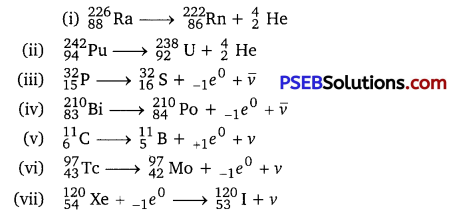Question 7.
A radioactive isotope has a half-life of T years. How long will it take the activity to reduce to (a) 3.125% (b) 1% of its original value?
Half-life of the radioactive isotope = T years
Original amount of the radioactive isotope = N0
(a) After decay, the amount of the radioactive isotope = N
It is given that only 3.125% of N0 remains after decay. Hence, we can write
$$\frac{N}{N_{0}}$$ = 3.125% = $$\frac{3.125}{100}=\frac{1}{32}$$
But $$\frac{N}{N_{0}}=e^{-\lambda t}$$
where, λ = Decay constant t = Time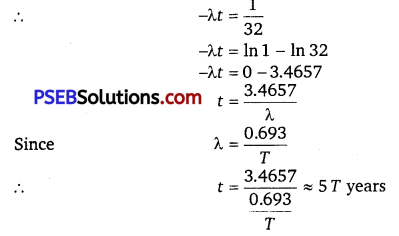Hence, the isotope will take about 5T years to reduce to 3.125% of its original value.

(b) After decay, the amount of the radioactive isotope = N
It is given that only 1% of N0 remains after decay. Hence, we can write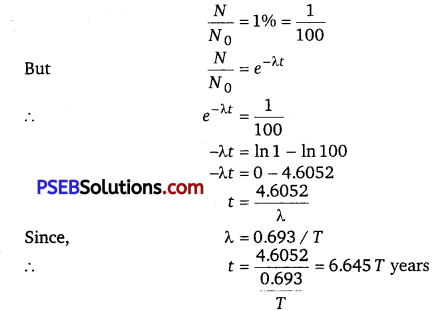Hence, the isotope will take about 6.645 T years to reduce to 1% of its original value.

Question 8.
The normal activity of living carbon-containing matter is found to be about 15 decays per minute for every gram of carbon. This activity arises from the small proportion of radioactive 614C present with the stable carbon isotope 614C. When the organism is dead, its interaction with the atmosphere (which maintains the above equilibrium activity) ceases and its activity begins to drop. From the known half-life (5730 years) of 612C, and the measured activity, the age of the specimen can be approximately estimated. This is the principle of dating 612C used in archaeology. Suppose a specimen from Mohenjodaro gives an activity of 9 decays per minute per gram of carbon. Estimate the approximate age of the Indus-Valley civilization.
Decay rate of living carbon-containing matter, R = 15 decays/min
Let N be the number of radioactive atoms present in a normal carbon-containing matter.
Half-life of 612C, T1/2 = 5730 years
The decay rate of the specimen obtained from the Mohenjodaro site
R’ = 9 decays/min
Let N be the number of radioactive atoms present in the specimen during the Mohenjodaro period.
Therefore, we can relate the decay constant, λ and time, t as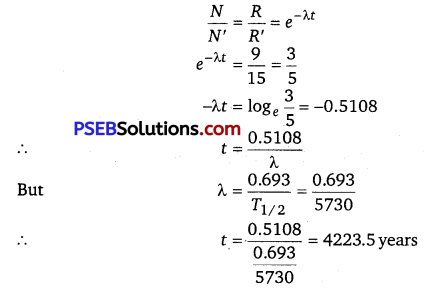Hence, the approximate age of the Indus-Valley civilization is 4223.5 years.

Question 9.
Obtain the amount of 2760Co necessary to provide a radioactive source of 8.0 mCi strength.
The half-life of 2760Co is 5.3 years.
The strength of the radioactive source is given as
$$\frac{d N}{d t}$$ = 8.0 mCi
= 8 x 10-3 x 3.7 x 1010
= 29.6 x 107 decay/s
where, N = Required number of atoms
Half-life of 2760Co, T1/2 = 5.3 years
= 5.3 x 365 x 24 x 60 x 60
= 1.67 x 108 s
For decay constant λ, we have the rate of decay as $$\frac{d N}{d t}$$ =λN
Where λ = $$\frac{0.693}{T_{1 / 2}}=\frac{0.693}{1.67 \times 10^{8}} \mathrm{~s}^{-1}$$
∴ N = $$\frac{1}{\lambda} \frac{d N}{d t}$$
= $$\frac{\frac{29.6 \times 10^{7}}{0.693}}{1.67 \times 10^{8}}$$ = 7.133 x 1016 atoms
For 27Co60
Mass of 6.023 x 1023 (Avogadro’s number) atoms = 60 g
∴ Mass of 7.133 x 1016 atoms = $$\frac{60 \times 7.133 \times 10^{16}}{6.023 \times 10^{23}}$$ = 7.106 x 10-6g
Hence, the amount of 27Co60 necessary for the purpose is 7.106 x 10-6g.

Question 10.
The half-life of 3890Sr is 28 years. What is the disintegration rate of 15 mg of this isotope?
Half-life of 3890Sr, t1/2 = 28 years
= 28 x 365 x 24 x 60 x 60
= 8.83 x 108s
Mass of the isotope, m = 15 mg
90 g of 3890Sr atom contains 6.023 x 1023 (Avogadro’s number) atoms.
Therefore 15 mg of 3890 Sr contains $$\frac{6.023 \times 10^{23} \times 15 \times 10^{-3}}{90}$$
i. e.,1.0038 x 1020 number of atoms
Rate of disintegration, = $$\frac{d N}{d t}=\lambda N$$
where, λ = Decay constant = $$\frac{0.693}{8.83 \times 10^{8}} \mathrm{~s}^{-1}$$
∴ $$\frac{d N}{d t}=\frac{0.693 \times 1.0038 \times 10^{20}}{8.83 \times 10^{8}}$$
= 7.878 x 1010 atoms/s
Hence, the disintegration rate of 15 mg of the given isotope is 7.878 x 1010 atoms/s.

Question 11.
Obtain approximately the ratio of the nuclear radii of the gold isotope 79197Au and the silver isotope 47107Ag.
Nuclear radius of the gold isotope 79197Au = RAu
Nuclear radius of the silver isotope 47107Ag =RAg
Mass number of gold, AAU = 197
Mass number of silver, AAg =107
The ratio of the radii of the two nuclei is related with their mass numbers as
$$\frac{R_{\mathrm{Au}}}{R_{\mathrm{Ag}}}=\left(\frac{A_{\mathrm{Au}}}{A_{\mathrm{Ag}}}\right)^{1 / 3}$$
= $$=\left(\frac{197}{107}\right)^{1 / 3}$$ = 1.2256
Hence, the ratio of the nuclear radii’of the gold and silver isotopes is about 1.23.Question 12.
Find the Q- value and the kinetic energy of the emitted α-particle in the a-decay of
(a) 88226 Ra and (b) 86220 Rn.
Given m (88226Ra) = 226.02540u, m (86222Rn) = 222.01750u,
m(86226Rn) = 220.01137 u, (84216Po) = 216.00189 u.
(a) Alpha particle decay of 88226Ra emits a helium nucleus. As a result, its
mass number reduces to (226 – 4) 222 and its atomic number reduces to (88 – 2) 86.
This is shown in the following nuclear reaction
88226Ra → 86222Rn+ 24He

Q-value of emitted α-particle
= (Sum of initial mass – Sum of final mass) c2
where c = speed of light It is given that
m (88226Ra) =226.02540 u
m (86222Rn) = 222.01750 u
m (24He) = 4.002603 u
Q-value =[226.02540 – (222.01750 + 4.002603)] u c2
= 0.005297 u c2
But 1 u = 931.5 MeV/c2
∴ Q = 0.005297 x 931.5 ≈ 4.94 MeV
I Mass number after decay
Kinetic energy of the α -particle = $$\left(\frac{\text { Mass number after decay }}{\text { Mass number before decay }}\right)$$ x Q
=$$\frac{222}{226}$$ x 4.94=4.85MeV ,

(b) Alpha particle decay of (86222Rn)
86222Rn + 84216Po + 24He
It is given that
Mass of (86220Rn) = 220.01137 u
Mass of (84216P0) = 216.00189 u
∴ Q-value =[220.01137 – (216.00189 + 4.002603)] x 931.5 ≈ 641 MeV
Kinetic energy of the α -particle = $$\left(\frac{220-4}{220}\right)$$ x 6.41 = 6.29 MeV

Question 13.
The radionuclide 11C decays according to 611C → 511B + e+ + v: T1/2 = 20.3 min
The maximum energy of the emitted positron is 0.960 MeV. Given the mass values.
m (611C) = 11.011434 u and (511B) = 11.009305 u,
calculate Q and compare it with the maximum energy of the positron emitted.
Mass difference Δm = mN(611C) – {mN(511B) + me}
where, mN denotes that masses of atomic nuclei.
If we take the masses of atoms, then we have to add 6me for 11C and 5me
for 11B, then Mass difference
= m(611C -6me) -{m(511B – 5me + me)}
= {m(11C)-m(611B)-2me}
= 11.011434 -11.009305 – 2 x 0.000548
= 0.001033 u
Q = 0.001033 x 931.5 MeV
= 0.962 MeV
This energy is nearly the same as energy carried by positron (0.960 MeV). The reason is that the daughter nucleus is too heavy as compared to e+ and v, so it carries negligible kinetic energy. Total kinetic energy is shared by positron and neutrino; here energy carried by neutrino (Ev) is minimum so that energy carried by positron (Ee) is maximum (practically, Ee ≈ Q).

Question 14.
The nucleus 1023Ne decays by β emission. Write down the β decay equation and determine the maximum kinetic energy of the electrons emitted. Given that: m (1023Ne) = 22.994466 u m (1123Na) = 22.089770 u
In β emission, the number of protons increases by 1, and one electron and an antineutrino are emitted from the parent nucleus. β emission of the nucleus 1023Ne
1023Ne → 1123Na +e+$$\bar{v}$$ +Q
It is given that
Atomic mass m of (1023Ne) = 22.994466 u
Atomic mass m of (1123 Na) = 22.089770 u
Mass of an electron, me = 0.000548 u
Q-value of the given reaction is given as
Q = [m(1023Ne)-{m(1123Na) + me}]c2
There are 10 electrons in 1023Ne and 11 electrons in 1123Na.
Hence, the mass of the electron is cancelled in the Q-value equation.
∴ Q = [22.994466 -22.089770] c2
= 0.004696 uc2
But 1 u = 981.5 MeV/c2
∴ Q = 0.004696 uc2 x 931.5 = 4.374 MeV
The daughter nucleus is too fifeavy as compared to e and $$\bar{v}$$.
Hence, it carries negligible energy. The kinetic energy of the antineutrino is nearly zero.
Hence, the maximum kinetic energy of the emitted electrons is almost equal to the Q-value, i. e., 4.374 MeV.

Question 15.
The Q value of a nuclear reaction A + b → C + d is defined by Q = [mA + mb -mc -md]c2
where the masses refer to the respective nuclei. Determine from the given data the Q-value of the following reactions and state whether the reactions are exothermic or endothermic.
(i) 11H + 13H → 12H + 12H
(ii) 612 C + 612 C → 1020Ne + 2He
Atomic masses are given to be
m (12H) = 2.014102 u
m(13H) = 3.016049 u
m(612C) = 12.000000 u
m(1020Ne) = 19.992439 u
(i) The given nuclear reaction is
11H + 13H → 12H + 12H
It is given that
Atomic mass m of (11H) = 1.007825 u
Atomic mass m of (13H) = 3.016049 u
Atomic mass m of (12H) = 2.014102 u

According to the question, the Q-value of the reaction can be written as
Q = [m (11H) + m (13H) -2m (12H)] c2
= [1.007825 + 3.016049 – 2 x 2.014102] c2
Q = (-0.00433 c2)u
But 1 u = 931.5MeV/c2
∴ Q = -0.00433 x 931.5 = -4.0334 MeV
The negative Q-value of the reaction shows that the reaction is endothermic.

(ii) The given nuclear reaction is
126C + 126C → 1020Ne + 24He
It is given that
Atomic mass m of (126C) = 12.0 u
Atomic mass m of (1020Ne) = 19.992439 u
Atomic mass m of (24He) = 4.002603 u
The Q-value of this reaction is given as
Q = [2m (126C) – m (1020Ne) – m (24He)] c2
= [2 x 12.0 -19.992439 – 4.002603] c2
= (0.004958 c2) u
But 1 u = 931.5 MeV/c2
Q = 0.004958 x 931.5 = 4.618377 MeV
The positive Q-value of the reaction shows that the reaction is exothermic.Question 16.
Suppose, we think of fission of a 2656Fe nucleus into two equal fragments, 1328Al. Is the fission energetically possible? Argue by working out Q of the process. Given m (2656Fe) = 55.93494 u and m(1328Al) = 27.98191 u.
The fission of 2656Fe can be given as
2656 Fe →2 1328Al
It is given that
Atomic mass m of (2656Fe) = 55.93494 u
Atomic mass m of (1328Al) = 27.98191 u
The Q-value of this nuclear reaction is given as
Q = [m (2656Fe) -2m (1328Al)] c2
= [55.93494 – 2 x 27.98191] c2
= (-0.02888 c2) u
Butl u = 931.5 MeV/c2
∴ Q =-0.02888 x 931.5 =-26.902 MeV
The Q-value of the fission is negative. Therefore, the fission is not possible energetically. For an energetically-possible fission reaction, the Q-value must be positive.

Question 17.
The fission properties of 94239Pu are very similar to those of 94239U. The average energy released per fission is 180 MeV. How much energy, in MeV, is released if all the atoms in 1 kg of pure 94239Pu undergo fission?
Average energy released per fission of 94239Pu, Eav = 180 MeV
Amount of pure 94Pu239, m = 1 kg = 1000 g
NA = Avogadro number = 6.023 x 1023
Mass number of 94239Pu = 239 g

1 mole of 94Pu239 contains NA atoms
∴ 1 kg of 94Pu239 contains $$\left(\frac{N_{A}}{\text { Mass number }} \times m\right)$$ atoms
= $$\frac{6.023 \times 10^{23}}{239} \times 1000$$ = 2.52 x 1024 atoms
∴ Total energy released during the fission of 1 kg of 94239 Pu is calculated as
E = Eav x 2.52 x1024
= 180 x 2.52 x 1024
= 4.536 x 1026 MeV
Hence, 4.536 x1026 MeV is released if all the atoms in 1 kg of pure 94Pu239 undergo fission.

Question 18.
A 1000 MW fission reactor consumes half of its fuel in 5.00 y. How much 92235U did it contain initially? Assume that the reactor operates 80% of the time, that all the energy generated arises from the fission of 92235U, and that this nuclide is consumed only by the fission process.
Half-life of the fuel of the fission reactor, t1/2 =5 years.
= 5 x 365 x 24 x 60 x 60 s
We know that in the fission of 1 g of 92235 U nucleus, the energy released is equal to 200 MeV.
1 mole, i. e., 235 g of 92235 U contains 6.023 x 1023 atoms.
∴ 1 g of 92235 U= $$\frac{6.023 \times 10^{23}}{235}$$ atoms

The total energy generated per gram of 92235U is calculated as
E= $$\frac{6.023 \times 10^{23}}{235} \times 200 \mathrm{MeV} / \mathrm{g}$$
= $$\frac{200 \times 6.023 \times 10^{23} \times 1.6 \times 10^{-19} \times 10^{6}}{235}$$
= 8.20 x 1010 J/g
The reactor operates only 80% of the time.

Hence, the amount of 92235 U consumed in 5 years by the 1000 MW fission
reactor is calculated as
= $$\frac{5 \times 80 \times 60 \times 60 \times 365 \times 24 \times 1000 \times 10^{6}}{100 \times 8.20 \times 10^{10}} \mathrm{~g}$$
≈1538 kg
∴ Initial amount of 92235U = 2 x 1538 = 3076 kg.

Question 19.
How long can an electric lamp of 100 W be kept glowing by fusion of 2.0 kg of deuterium? Take the fusion reaction as The given fusion reaction is 12H + 12H → 23He+n +3.27 MeV
The given fusion reaction is
12H + 12H → 23He+n +3.27 MeV
Amount of deuterium, m = 2 kg
1 mole, i.e., 2 g of deuterium contains 6.023 x 1023 atoms.
∴ 2.0 kg of deuterium contains = $$\frac{6.023 \times 10^{23}}{2} \times 2000$$
= 6.023 x 10 26 atoms

It can be inferred from the given reaction that ‘when two atoms of deuterium fuse, 3.27 MeV energy is released. ” ‘
∴ Total energy per nucleus released in the fusion reaction
E = $$\frac{3.27}{2} \times 6,023 \times 10^{26} \mathrm{MeV}$$
= $$\frac{3.27}{2} \times 6.023 \times 10^{26} \times 1.6 \times 10^{-19} \times 10^{6}$$
= 1.576 x 1014 J
Power of the electric lamp, P = 100 W = 100 J/s
Hence, the energy consumed by the lamp per second = 100 J
The total time for which the electric lamp will glow is calculated as $$\frac{1.576 \times 10^{14}}{100 \times 60 \times 60 \times 24 \times 365}$$
≈ 4.9 x 104 years

Question 20.
Calculate the height of the potential harrier for a head-on collision of two deuterons. (Hint: The height of the potential barrier is given by the Coulomb repulsion between the two deuterons when they just touch each other. Assume that they can be taken as hard spheres of radius 2.0 fm).
When two deuterons collide head-on, the distance between their centres, d is given as Radius of 1st deuteron + Radius of 2 nd deuteron
Radius of a deuteron nucleus = 2 fm =2 x 10-15 m
∴ d = 2x 10-15 +2 x 10-15
=4 x 10-15 m
Charge on a deuteron nucleus = Charge on an electron = e =1.6 x 10-19C
Potential energy of the two-deuteron system
V = $$\frac{e^{2}}{4 \pi \varepsilon_{0} d}$$
where, $$\varepsilon_{0}$$ = permittivity of free space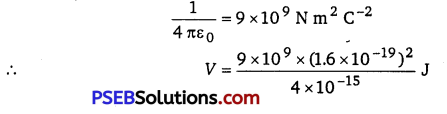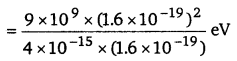= 360 keV
Hence, the height of the potential barrier of the two-deuteron system is 360 keV.

Question 21.
From the relation R = R0A1/3, where R0 is a constant and A is the mass number of a nucleus, show that the nuclear matter density is nearly constant (i. e., independent of A).
We have the expression for nuclear radius as
R=R0A1/3
where, R0 = Constant.
Nuclear matter density, ρ = $$\frac{\text { Mass of the nucleus }}{\text { Volume of the nucleus }}$$
Let m be the average mass of the nucleus.
Hence, mass of the nucleus = mA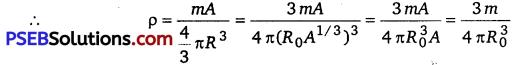Hence, the nuclear matter density is independent; of A. It is nearly constant.

Question 22.
For the β+ (positron) emission from a nucleus, there is another competing process known as electron capture (electron from an inner orbit, say, the K-shell, is captured by the nucleus, and a neutrino is emitted.)
e+ + AZ X → z-1AY+ u
Show that if β+ emission is energetically allowed, electron capture is necessarily allowed but not vice-Versa.
Let the amount of energy released during the electron capture process be Q1.
The nuclear reaction can be written as
e+ + AZ X → z-1Y+ v+ Q1 …………..(1)
Let the amount of energy released during the positron capture process be Q2.
The nuclear reaction can be written as
e+ + AZ X → z-1Y+e++ v+ Q2 ……………………..(2)
mN (zAX) = Nuclear mass of zA X
mN (z-1AY) = Nuclear mass of z-1AY
m(ZA
X) = Atomic mass of ZA X
m (z-1A Y) = Atomic mass of z-1AY
me = Mass of an electron
c = Speed of light
Q-value of the electron capture reaction is given as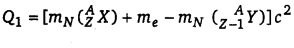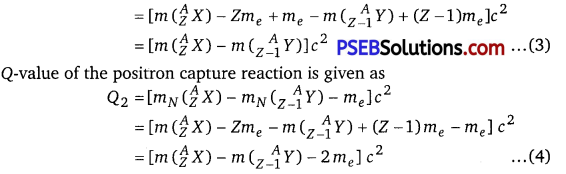It can be inferred that if Q2 > 0, then Q1 > 0; Also, if Q1> 0, it does not necessarily mean that Q2 > 0.
In other words, this means that if β+ emission is energetically allowed, then the electron capture process is necessarily allowed, but not vice-versa. This is because the Q-value must be positive for an energetically-allowed nuclear reaction.Question 23.
In a periodic table the average atomic mass of magnesium is given as 24.312 u. The average value is based on their relative natural abundance on earth. The three isotopes and their masses are 1224 Mg (23.98504 u), 1225Mg (24.98584 u) and 1226Mg (25.98259 u). The natural abundance of 1224Mg is 78.99% by mass. Calculate the abundances of other two isotopes.
Average atomic mass of magnesium, m = 24.312 u
Mass of magnesium 1224Mg isotope, m1 = 23.98504 u
Mass of magnesium 1225Mg isotope, m2 = 24.98584 u
Mass of magnesium 1226 Mg isotope, m3 = 25.98259 u
Abundance of 1224Mg, n1 = 78.99%
Abundance of 1225Mg, n2 = x%
Hence, abundance of 1226 Mg, n3 = 100 – x- 78.99% = (21.01 – x)%

We have the relation for the average atomic mass as
m = $$\frac{m_{1} n_{1}+m_{2} n_{2}+m_{3} n_{3}}{n_{1}+n_{2}+n_{3}}$$
243.12 = $$\frac{23.98504 \times 78.99+24.98584 \times x+25.98259 \times(21.01-x)}{100}$$
2431.2 = 1894.5783096 + 24.98584x + 545.8942159- 25.98259 x
0.99675x =9.2725255
∴ x ≈ 9.3%
and 21.01-x =11.71%
Hence, the abundance of 1225Mg is 9.3% and that of 1226 Mg is 11.71%.

Question 24.
The neutron separation energy is defined as the energy required to remove a neutron from the nucleus.
Obtain the neutron separation energies of the nuclei 2041Ca and 1327Al from
the following data:
m(2040Ca) = 39-962591u
m (2041 Ca) = 40.962278 u
m (2613Al) = 25.986895 u
m (2713Al) = 26.981541 u
For 2041Ca : Separation energy = 8.363007 MeV
For 2713 A1: Separation energy = 13.059 MeV
(on1) is removed from a 2041Ca.

Thus, the corresponding nuclear reaction can be written as
2041Ca → 2040Ca + 01n
It is given that
m(2040Ca) = 39.962591 u
m(2041Ca )= 40.962278 u
m(on1) = 1.008665 u
The mass defect of this reaction is given as
Δm = m(2040Ca) + (01n )-m(2041Ca)
= 39.962591 +1.008665 – 40.962278
= 0.008978 u

But 1 u = 931.5 MeV/c2
∴ Δm = 0.008978×931.5 MeV/c2
Hence, the energy required for neutron removal is calculated as
E = Δmc2
= 0.008978 x 931.5 = 8.363007 MeV
For 1327 Al, the neutron removal reaction can be written as
1327Al → 1326 Al + 01n
It is given that
m (1327Al) = 26.981541 u
m (1326 Al) = 25.986895 u
The mass defect of this reaction is given as
Δm = m (1326 Al) + m (01n) – m (1327 Al)
= 25.986895 +1.008665 – 26.981541
= 0.014019 u

But 1 u = 931.5 MeV/c2
∴ Δm = 0.014019 x 931.5 MeV/c2
Hence, the energy required for neutron removal is calculated as
E = Δmc2
= 0.014019 x 931.5 = 13.059 MeV

Question 25.
A source contains two phosphorous radio nudides 1532P (TM1/2 =14.3d)
and 1533P (T1/2 =253d). Initially, 10% of the
decays come from 1533P. How long one must wait until 90% do so?
Let radionuclide be represented as P1 (T1/2 =14.3 days) and
P2(T1/2 = 25.3 days).
Initial decay is 90% from P1 and 10% from P2. With the passage of rime,
amount of P1 will decrease faster than that of P2.

As rate of disintegration ∝ N or mass M. Initial ratio of P1 to P2 is 9: 1. Let mass of P1 be 9x and that of P2 be x. Let after t days mass of P1 become y and that of P2 become 9y.
Using half-life formula, $$\frac{M}{M_{0}}=\left(\frac{1}{2}\right)^{n}$$ , where n is number of half lives,
n = $$\frac{t}{T_{1}}$$
$$\frac{y}{9 x}=\left(\frac{1}{2}\right)^{n_{i}}$$ ……………………. (1)
Where, n1 = $$\frac{t}{T_{2}}$$
$$\frac{9 y}{x}=\left(\frac{1}{2}\right)^{n_{2}}$$ …………………………… (2)
On dividing eq.(1) by eq(2), we get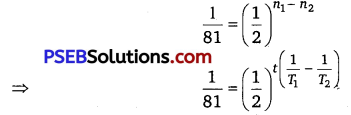log 1- log 81 = t$$\left(\frac{1}{T_{1}}-\frac{1}{T_{2}}\right)$$ $$(\log 1-\log 2)$$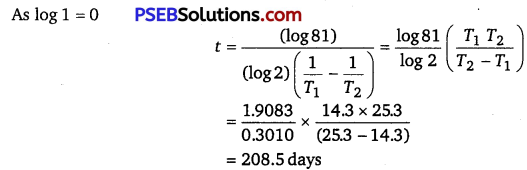Question 26.
Under certain circumstances, a nucleus can decay by emitting a particle more massive than an a-particle. Consider the following decay processes:
88223Ra → 82209Pb+ 614C
88223Ra → 86219Rn + 24He
Calculate the Q-values for these decays and determine that both are energetically allowed.
Take a 614C emission nuclear reaction
88223Ra → 82209Pb+ 614C
We know that
Mass of 88223Ra, m1 = 223.01850 u
Mass of 82209 Pb, m2 = 208.98107 u
Mass of 614C, m3 = 14.00324 u
Hence, the Q-value of the reaction is given as
Q = (m1-m2-m3‘)c2
= (223.01850 -208.98107-14.00324)c2
= (0.03419 c2)u
But 1u = 931.5 MeV/c2
∴ Q = 0.03419 x 931.5 = 31.848 MeV

Hence, the Q-value of the nuclear reaction is 31.848 MeV. Since the value is positive, the reaction is energetically allowed.
Now take a 24He emission nuclear reaction
88223Ra → 86219Rn + 24He
We know that
Mass of 88223Ra, m1 = 223.01850
Mass of 86219Rn, m2 = 219.00948
Mass of 24He, m3 = 4.00260
Q-value of this nuclear reaction is given as
Q = (m1-m2-m3)c2
= (223.01850 -219.00948-4.00260)c2
= (0.00642 c2)u
= 0.00642 x 931.5 = 5.98 MeV
Hence, the Q value of the second nuclear reaction is 5.98 MeV. Since the value is positive, the reaction is energetically allowed.Question 27.
Consider the fission of 92238U by fast neutrons. In one fission event, no neutrons are emitted and the final end products, after the beta decay of the primary fragments, are 58140Ce and 4499Ru.
Calculate Q for this fission process. The relevant atomic and particle masses are .
m = ( 92238U) = 238.05079 u
m = ( 58140Ce) = 139.90543 u
m = ( 4499Ru) = 98.90594 u
In the fission of 92238U, 10β particles decay from the parent nucleus. The nuclear reaction can be written as
92238U +01n → 58140Ce+4499Ru+10-10e

It is given that
Mass of a nucleus, m1(92238U) = 238.05079 u
Mass of a nucleus, m2(58140Ce) = 139.90543 u
Mass of a nucleus,m3 (4499Ru) = 98.90543 u
Mass of a neutron,m4 (01n) = 1.008665 u
Q-value of the above equation,
Q = [m'(92238U) + m(01n) – m'(58140Ce) – m'(4499Ru) -10 me]c2
Where, m’ represents the corresponding atomic masses of the nuclei,
m'(92238U) = m1 – 92 me
m’ (58140Ce) = m2 – 58me
m’ (4499Ru) = m2 – 44 me
m(01n) = m4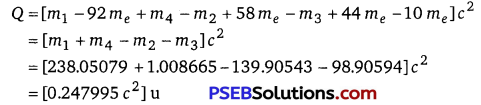But 1u = 931.5 MeV/c2
∴ Q = 0.247995 x 931.5 = 231.007 MeV
Hence, the Q-value of the fission process is 231.007 MeV.

Question 28.
Consider the D-T reaction (deuterium-tritium fusion)
12H +H 1324He + n
(a) Calculate the energy released in MeV in this reaction from the data
m (12H) = 2.014102 u
m(H 13) = 3.016049 u

(b) Consider the radius of both deuterium and tritium to be approximately 2.0 fm. What is the kinetic energy needed to overcome the coulomb repulsion between the two nuclei? To what temperature must the gas be heated to initiate the reaction? (Hint: Kinetic energy required for one fusion event = average thermal kinetic energy available with the interacting particles =2(3kT/2);k = Boltzman’s constant, T = absolute temperature.)
(a) Take the D-T nuclear reaction :
12H +H 1324He + n
It is given that
Mass of 12H, m1 = 2.014102 u
Mass of H 13, m2 = 3.016049 u
Mass of 24He, m3 = 4.002603 u
Mass of 01n, m4 = 1.008665 u
Q-value of the given D-T reaction is
Q = [m1 + m2 – m3 – m4]c2
= [2.014102 + 3.016049 – 4.002603 -1.008665]c2
= [0.018883 c2]u
But 1 u = 931.5 MeV/c2
∴ Q = 0.018883 x 931.5 = 17.59 MeV

(b) Radius of deuterium and tritium, r ≈ 2.0 fm = 2 x 10-15 m
Distance between the two nuclei at the moment when they touch each other,
d = r + r = 4 x 10-15 m
Charge on the deuterium nucleus = e
Charge on the tritium nucleus = e
Hence, the repulsive potential energy between the two nuclei is given as
V = $$\frac{e^{2}}{4 \pi \varepsilon_{0}(d)}$$
Where, $$\varepsilon_{0}$$ = Permittivity of free space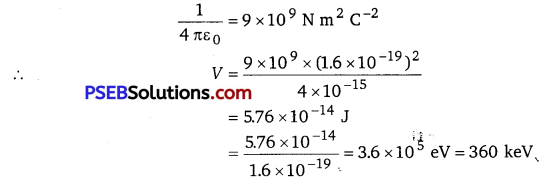Hence, 5.76 x 10-14 J or 360 key of kinetic energy (KE) is needed to overcome the Coulomb repulsion between the two nuclei.
However, it is given that
K.E = 2 x $$\frac{3}{2}$$ kt
where, k = Boltzmann constant = 1.38 x 10-23 m2 kg s-2 K-1
T = Temperature required for triggering the reaction
T = $$\frac{K E}{3 K}$$
= $$\frac{5.76 \times 10^{-14}}{3 \times 1.38 \times 10^{-23}}$$
= 1.39 x 109 K
Hence, the gas must be heated to a temperature of1.39 x 109 K to initiate the reaction.

Question 29.
Obtain the maximum kinetic energy of β-particles, and the radiation frequencies of y decays in the decay scheme shown in Fig. 13.6. You are given that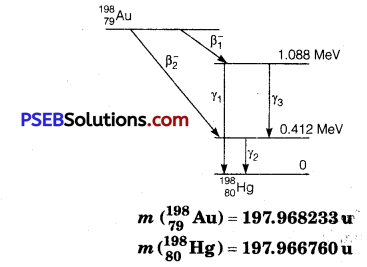It can be observed from the given γ-decay diagram that γ1 decays from 1.088 MeV
energy level to the O’MeV energy level.
Hence, the energy corresponding to γ1-decay is given as
E1 =1.088-0 =1.088 MeV
hv1 = 1.088 x 1.6 x 10-19 x 106
where, h = Planck’s constant = 6.63 x 10-34 Js
∴ v1 = $$\frac{E_{1}}{h}$$
= $$\frac{1.088 \times 1.6 \times 10^{-19} \times 10^{6}}{6.63 \times 10^{-34}}$$
= 2.637 x 1020 Hz
It can be observed from the given γ-decay diagram that γ2 decays from 0.412 MeV energy level to the 0 MeV energy level.
Hence, the energy corresponding to γ2-decay is given as :
E2 =0.412-0 =0.412 MeV
hv2 = 0.412 x 1.6 x 10-19 x 106 J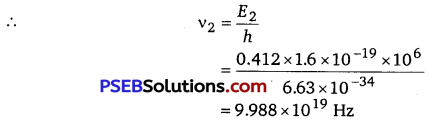It can be observed from the given γ-decay diagram that γ2 decays from the 1.088 MeV energy level to the 0.412 MeV energy level.
Hence, the energy corresponding to γ3 -decay is given as
E3 =1.088- 0.412 = 0.676 MeV
hv3 = 0.676 x 1.6 x 10 -19 J

∴ v3 = $$\frac{E_{3}}{h}=\frac{0.676 \times 1.6 \times 10^{-19} \times 10^{6}}{6.63 \times 10^{-34}}$$
= 1.639 x 1020 Hz
Mass of m (79198 Au) = 197.968233 u
Mass of m (80198Hg) = 197.966760 u
1 u = 931.5 MeV/c2
Energy of the highest level is given as
E =[ (79198 Au) – m (80198Hg)]
= 197.968233 -197.966760
= 0.001473 u
= 0.001473 x 931.5 = 1.3720995 MeV
β1 decays from the 1.3720995 MeV level to the 1.088 MeV level
Maximum kinetic energy of the β1 particle = 1.3720995 – 1.088
= 0.2840995 MeV
β2 decays from the 1.3720995.MeV level to the 0.412 MeV level ,
∴ Maximum kinetic energy of the β2 particle = 1.3720995-0.412
= 0.9600995 MeV

Questions 30.
Calculate and compare the energy released by
(a) fusion of 1.0 kg of hydrogen deep within Sun and
(b) the fission of 1.0 kg of 92235U in a fission reactor.
(a) Amount of hydrogen, m = 1 kg = 1000 g
1 mole, i. e, 1 g of hydrogen (11H) contains 6.023 x 1023 atoms.
∴ 1000 g of 11H contains 6.023 x 1023 x 1000 atoms.
Within the sun, four 11H nuclei combine and form one 24He nucleus. In this process 26 MeV of energy is released.
Hence, the energy released from the fusion of 1 kg 11H is
E1 = $$\frac{6.023 \times 10^{23} \times 26 \times 10^{3}}{4}$$
= 39.1495 x 1026MeV

(b) Amount of 92235U = 1 kg = 1000 g
1 mole, i. e., 235 g of 92235U contains 6.023 x 1023 atoms.
∴1000 g of 92235U contains $$\frac{6.023 \times 10^{23} \times 1000}{235}$$atoms
It is known that the amount of energy released in the fission of one atom of 92235U is 200 MeV.
Hence, energy released from the fission of 1 kg of 92235U is
E2 = $$\frac{6.023 \times 10^{23} \times 1000 \times 200}{235}$$
= 5.106 x 1026 MeV
∴ $$\frac{E_{1}}{E_{2}}=\frac{39.1495 \times 10^{26}}{5.106 \times 10^{26}}$$ = 7.67 ≈ 8
Therefore, the energy released in the fusion of 1 kg of hydrogen is nearly 8 times the energy released in the fission of 1 kg of uranium.Question 31.
Suppose India had a target of producing by 2020 AD, 200,000 MW of electric power, ten percent of which was to be obtained from nuclear power plants. Suppose we are given that, on an average, the efficiency of utilization (i. e., conversion to electric energy) of thermal energy produced in a reactor was 25%. How much amount of fissionable uranium would our country need per year by 2020? Take the heat energy per fission of 235U to be about 200 MeV.
Amount of electric power to be generated, P = 2 x105 MW
10% of this amount has to be obtained from nuclear power plants.
P1 = $$\frac{10}{100}$$ x 2 x 105
∴ Amount of nuclear power,
= 2 x 104 MW
= 2x 104 x 106 J/s
= 2 x 1010 x 60 x 60 x 24 x 365 J/y
Heat energy released per fission of a 235 U nucleus, E = 200 MeV
Efficiency of a reactor = 25%
Hence, the amount of energy converted into the electrical energy per fission is calculated as
$$\frac{25}{100} \times 200=50 \mathrm{MeV}=50 \times 1.6 \times 10^{-19} \times 10^{6}=8 \times 10^{-12} \mathrm{~J}$$
Number of atoms required for fission per year
$$\frac{2 \times 10^{10} \times 60 \times 60 \times 24 \times 365}{8 \times 10^{-12}}$$ = 78840 x 1024 atoms
1 mole, i. e., 235 g of U235 contains 6.023 x 1023 atoms.
∴ Mass of 6.023 x 1023 atoms of U235 = 235 g = 235 x 10-3 kg
∴ Mass of 78840×1024 atoms of U235 = $$\frac{235 \times 10^{-3}}{6.023 \times 10^{23}} \times 78840 \times 10^{24}$$
= 3.076 x104 kg
Hence, the mass of uranium needed per year is 3.076 x 104 kg.